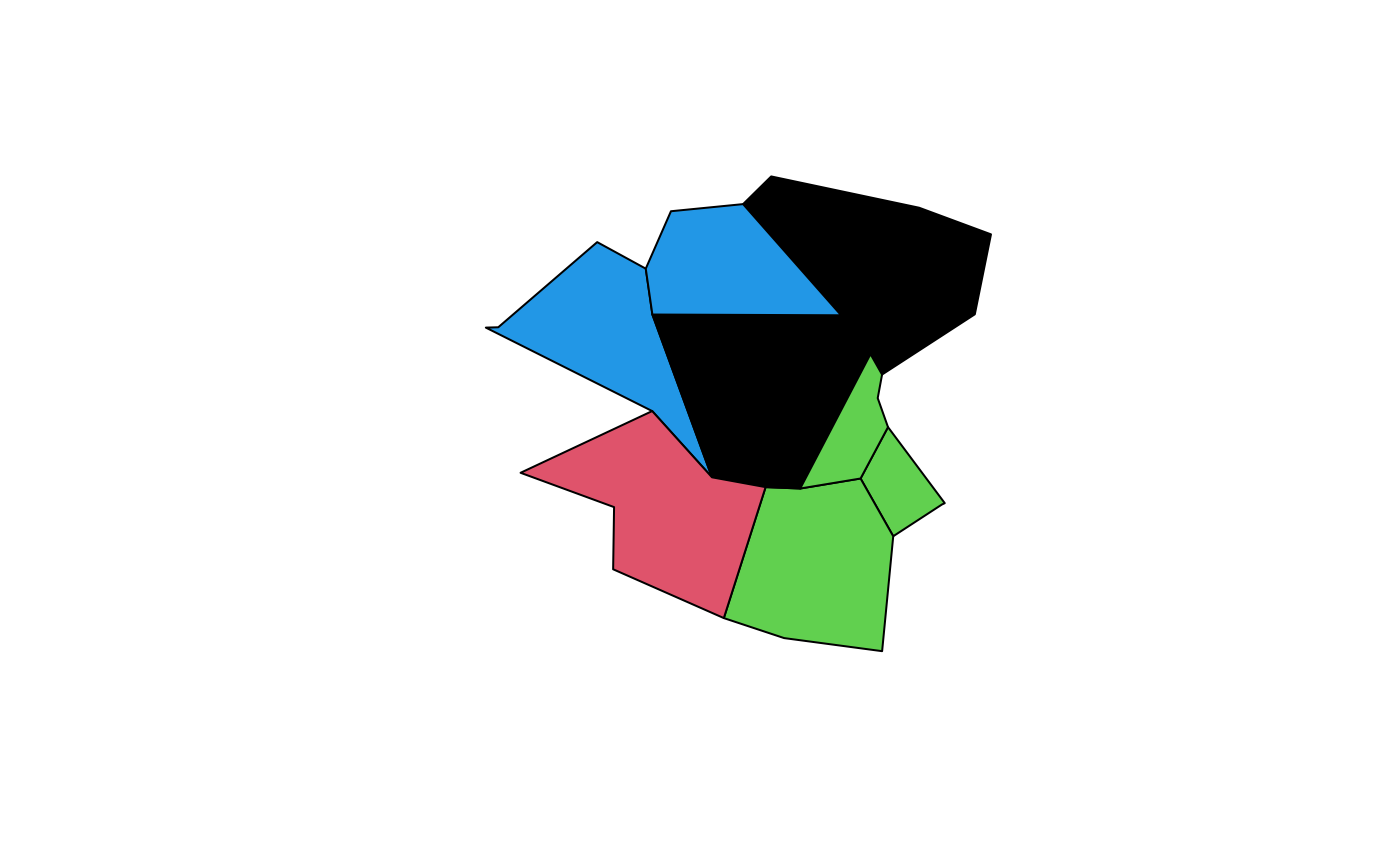Returns a character vector of NE, SE, SW, NW corresponding to north-east, south-east quadrants respectively. If number_out is TRUE, returns numbers from 1:4, respectively.

## Usage

``quadrant(x, cent = NULL, number_out = FALSE)``

## Arguments

x

Object of class sf

cent

The centrepoint of the region of interest. Quadrants will be defined based on this point. By default this will be the geographic centroid of the zones.

number_out

Should the result be returned as a number?

Other geo: `bbox_scale()`, `geo_bb_matrix()`, `geo_bb()`
``````x = zones_sf